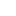v2.26ICurve3D Interface Reference

Represents a generic curve in three-dimensional space. A generic curve is a vector function of a scalar parameter defined on some interval of parameter values. More...

Inheritance diagram for ICurve3D:## Public Member Functions

HRESULT GetCopy ([out, retval] ICurve3D **ppCurve3D)
Returns a copy of the curve.

HRESULT GetBeginPoint ([out, retval] Point3D *pPoint3D)
Calculates the start point of the curve.

HRESULT GetEndPoint ([out, retval] Point3D *pPoint3D)
Calculates the end point of the curve.

HRESULT GetPointOn ([in] double param, [out, retval] Point3D *pPoint3D)
Calculates a point on the curve by the given parameter value. More...

HRESULT GetLength ([out, retval] double *pLength)
Calculates the metric length of the curve.

HRESULT GetGabarit ([out, retval] Cube *pCube)
Calculates the bounding box of a curve.

HRESULT IsClosed ([out, retval] VARIANT_BOOL *pResult)
Checks if the curve is closed.

HRESULT GetParameterAtDistance ([in] double startT, [in] double distance, [in] int direction, [out, retval] double *pResultT)
Calculates a point on the curve which is offset from a given reference point on the curve. More...

HRESULT GetTrimmed ([in] double t1, [in] double t2, [in] int sense, [out, retval] ICurve3D **ppTrimmedCurve)
Constructs a trimmed curve. More...

HRESULT PointProjection ([in] Point3D *point, [out, retval] double *pResultT)
Calculates the nearest projection of a point onto the curve. More...

HRESULT GetInterfaceByName ([in] BSTR interfaceName, [out, retval] IDispatch **ppInterface)
Returns an interface by its name. More...

HRESULT GetTransformed ([in] ITransform3D *pTransfrom, [out, retval] ICurve3D **ppTransformedCurve)
Returns curve copy transformed according to a given transform.

HRESULT GetOffseted ([in] Vector3D *pOffset, [out, retval] ICurve3D **ppOffsetCurve)
Returns a curve offset on the given vector.

## Properties

Curve3DType Curve3DType` [get]`
Returns the type of the curve.

double MinParameter` [get]`
The lower bound of the parameter interval the curve is defined on.

double MaxParameter` [get]`
The upper bound of the parameter interval the curve is defined on.

## Detailed Description

Represents a generic curve in three-dimensional space. A generic curve is a vector function of a scalar parameter defined on some interval of parameter values.

## ◆ GetInterfaceByName()

 HRESULT ICurve3D::GetInterfaceByName ( [in] BSTR interfaceName, [out, retval] IDispatch ** ppInterface )

Returns an interface by its name.

This method is provided as an alternative for the standard COM QueryInterface() method for cases where IUnknown is not accessible, like interoperating with the API from some interpreted languages.

Parameters
 [in] interfaceName The name of the requested interface. [out] ppInterface The requested interface.

## ◆ GetParameterAtDistance()

 HRESULT ICurve3D::GetParameterAtDistance ( [in] double startT, [in] double distance, [in] int direction, [out, retval] double * pResultT )

Calculates a point on the curve which is offset from a given reference point on the curve.

If the curve section from the reference point to the corresponding end point is shorter than the required offset distance, the calculation is performed on extension of the curve if it is possible to construct such extension. Otherwise the operation will fail.

Parameters
 [in] startT The parameter value characterizing the reference point. [in] distance The offset distance along the curve. [in] direction The offset direction. A non-negative value means the offset is done towards the increase of the parameter; Otherwise it is done towards the decrease of the parameter. [out] pResultT The parameter value of the resulting point.

## ◆ GetPointOn()

 HRESULT ICurve3D::GetPointOn ( [in] double param, [out, retval] Point3D * pPoint3D )

Calculates a point on the curve by the given parameter value.

If the parameter value falls outside the curve bounds defined by BeginParameter() and EndParameter(), the parameter is clamped to the nearest valid value.

Parameters
 [in] param Curve parameter. [out] pPoint3D The point on the curve.

## ◆ GetTrimmed()

 HRESULT ICurve3D::GetTrimmed ( [in] double t1, [in] double t2, [in] int sense, [out, retval] ICurve3D ** ppTrimmedCurve )

Constructs a trimmed curve.

Constructs a trimmed curve, with starting point corresponding to parameter value t1 and the end point corresponding to parameter value t2.

Direction of the constructed curve relative to the original curve may be changed by the 'sense' parameter.

For a closed curve it is possible to obtain a trimmed curve which passes through the start of the original curve.

For a closed or periodic curve, the three parameters 'sense', t1 and t2 unambiguously define the result.

In case of an unclosed curve, 'sense' and trimming bounds should correspond to each other: if sense = 1, then t1 < t2, if sense = -1, then t1 > t2,

If there is a discrepancy between 'sense' and trimming bounds, then the 'sense' parameter has a higher priority.

If parameters t1 and t2 are equal and the curve is closed, the resulting curve is closed.

Parameters
 [in] t1 Parameter value corresponding to start of the trimmed curve. [in] t2 Parameter value corresponding to end of the trimmed curve. [in] sense Direction of the trimmed curve relative to the original curve. 1 means the direction does not change, and -1 means the direction is changed to the opposite. [out] ppTrimmedCurve The constructed trimmed curve.

## ◆ PointProjection()

 HRESULT ICurve3D::PointProjection ( [in] Point3D * point, [out, retval] double * pResultT )

Calculates the nearest projection of a point onto the curve.

Finds the nearest point projection on the curve by a given initial approximation using the Newton method.

Parameters
 [in] point The given approximation point. [out] pResultT The parameter of a curve corresponding to the nearest projection point.